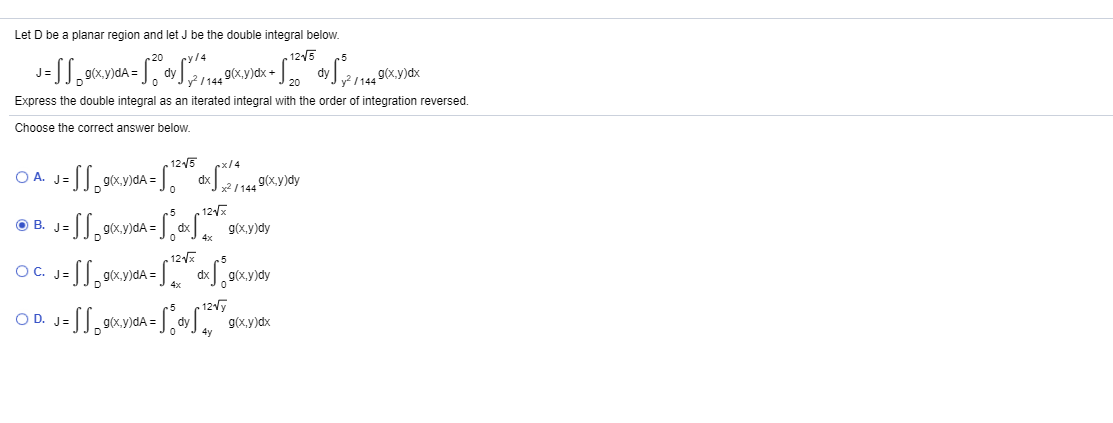# Let D be a planar region and let J be the double integral below. cy/4 „ 125 20 g(x.y)dx g(x.y)dx 20 Express the double integral as an iterated integral with the order of integration reversed. Choose the correct answer below. . 1215 rx/4 O A. dx x / 144 9(x.y)dy .5 12x B. J= g(x,y)dA = 12 5 OC. O C. J= 9(x.y)dA =. g(x,y)dy .5 - 12y O D. J= ty g(x,y)dx 4y

Question

It is on the study plan please ı need help urgentlyhelp_outlineImage TranscriptioncloseLet D be a planar region and let J be the double integral below. cy/4 „ 125 20 g(x.y)dx g(x.y)dx 20 Express the double integral as an iterated integral with the order of integration reversed. Choose the correct answer below. . 1215 rx/4 O A. dx x / 144 9(x.y)dy .5 12x B. J= g(x,y)dA = 12 5 OC. O C. J= 9(x.y)dA =. g(x,y)dy .5 - 12y O D. J= ty g(x,y)dx 4y fullscreen

### Want to see this answer and more?

Experts are waiting 24/7 to provide step-by-step solutions in as fast as 30 minutes!*

*Response times may vary by subject and question complexity. Median response time is 34 minutes for paid subscribers and may be longer for promotional offers.
Tagged in
Math
Calculus

### Other